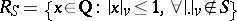# S-integer

As a simple example, let $S = \{ p _ { 1 } , \dots , p _ { n } \}$ be a finite set of rational prime numbers. The rational integers $a / b$, $a , b \in \mathbf{Z}$, relatively prime (cf. also Mutually-prime numbers), such that the set of prime divisors of $b$ (possibly empty) is contained in $S$ are the so-called $S$-integers (corresponding to the specific set $S$). Clearly, this is a subring $R_S$ of $\mathbf{Q}$. Let $R _ { S } ^ { * }$ denote the group of units of $R_S$, i.e. the group of multiplicatively invertible elements of $R_S$ (the $S$-units). Clearly, these are $\pm$ and the rational numbers $x$ in the prime decomposition of which only prime numbers from the set $S$ appear.

These notions can be defined in a more sophisticated way, the advantage of which is that it can be generalized to the more general case of a number field. For this the notion of absolute value on a number field is needed. Unfortunately, there is no general agreement on the definition of this notion. Below, this "absolute value" is taken in the sense of a metric as in [a1], Chap. 1, Sect. 4; Chap. 4, Sect. 4; equivalently, an absolute value is a function $\rho ^ { v(.) }$, where $\rho$ is a fixed, conveniently chosen positive real number $< 1$ and $v$ is a valuation, as defined and used in [a2], Chap. 1, § 2; Chap. 3 § 1, (cf. also Valuation, which gives a slightly different definition).

In the special case above, every rational prime number $p$ gives rise to a $p$-adic absolute value and all possible absolute values of $\mathbf{Q}$ are (up to topological equivalence) the $p$-adic ones (non-Archimedean), denoted by $|.|_p$, and the usual absolute value (Archimedean), denoted by $|.| _ { \infty }$. Let $M _ { \operatorname{Q} }$ denote the set of absolute values (more precisely, the set of equivalence classes of absolute values (i.e. places) of $\mathbf{Q}$; cf. also Place of a field). Thus, every element of this set is of the form $|.| v$, where $v$ is either a rational prime number or the symbol $\infty$. One now modifies the definition of the set $S$ above as the subset of $M _ { \operatorname{Q} }$ containing the absolute values (i.e. places) $|.| v$, where $v \in \{ p _ { 1 } , \dots , p _ { n } , \infty \}$. Thenand $R _ { S } ^ { * } = \{ x \in \mathbf{Q} : | x | _ { v } = 1 , \forall | \cdot | _ { v } \notin S \}$.

Consider now the more general situation, where a number field $K$ is taken in place of $\mathbf{Q}$ and its ring of integers $\mathcal{O} _ { K }$ is taken in place of $\bf Z$. Let $M _ { K }$ be the set of absolute values of $K$ (more precisely, the set of equivalence classes of absolute values, i.e. places, of $K$). These are divided into two categories, namely, the non-Archimedean ones, which are in one-to-one correspondence with the prime ideals (or, what is essentially the same, with the prime divisors) of $K$ and the Archimedean ones, which are in one-to-one correspondence with the isomorphic embeddings $K \hookrightarrow \bf C$ (complex-conjugate embeddings giving rise to the same absolute value). As before, let $S$ be a finite subset of $M _ { K }$ containing all Archimedean valuations of $K$. Then, the set $R_S$ of $S$-integers and the set $R _ { S } ^ { * }$ of $S$-units are defined exactly as in the case of rational numbers (see the definitions above), where now $\mathbf{Q}$ is replaced by $K$.

Many interesting problems concerning the solution of Diophantine equations are reduced to questions about $S$-integers of "particularly simple form" (e.g. linear forms in two unknown parameters), which are $S$-units, and then results are obtained by applying a variety of relevant results on $S$-integers and $S$-units.

How to Cite This Entry:
S-integer. Encyclopedia of Mathematics. URL: http://encyclopediaofmath.org/index.php?title=S-integer&oldid=50689
This article was adapted from an original article by N. Tzanakis (originator), which appeared in Encyclopedia of Mathematics - ISBN 1402006098. See original article